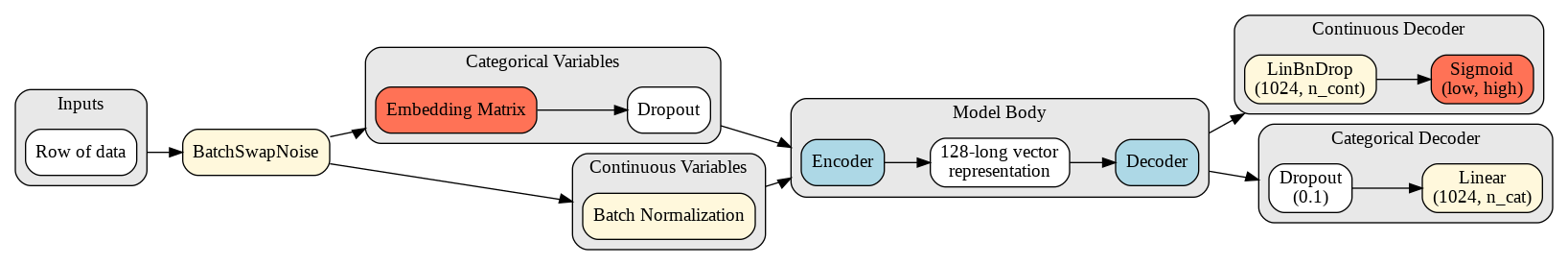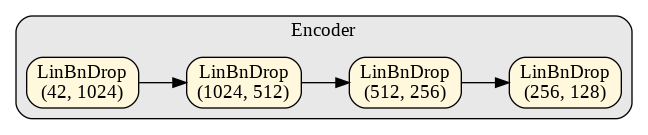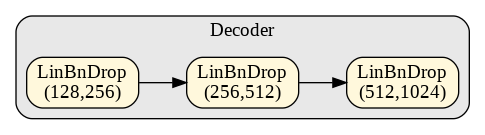A usage example and explaination of AutoEncoders in fastai
/mnt/d/jupyter/anaconda3/envs/nbagile/lib/python3.7/site-packages/fastprogress/fastprogress.py:102: UserWarning: Couldn't import ipywidgets properly, progress bar will use console behavior
warn("Couldn't import ipywidgets properly, progress bar will use console behavior")


This notebook is largely based on the fantastic work of Etienne Tremblay and what came from experiments as noted here

This article is also a Jupyter Notebook available to be run from the top down. There will be code snippets that you can then run in any environment.

Below are the versions of fastai and fastcore currently running at the time of writing this:

• fastai: 1.0.61
• fastcore: 1.0.16

## AutoEncoders, just what are they?

The problem: we have far too many input variables (200+).

Rather than trying to make a tabular model with all these features, we can compress them down via a technique called AutoEncoding.

Essentially we train a model whose sole purpose is to recreate the original input data!

But where does the reduction happen? Let's look at a model visualization of an auto-encoder, specifically the one we will be making!

### The Overall Model:The base of this model is extremely similar to fastai's TabularModel, minus a few distinctions:

• Our inputs immediatly pass through a BatchSwapNoise module, based on the Porto Seguro Winning Solution which inputs random noise into our data for variability
• After going through the embedding matrix the "layers" of our model include an Encoder and Decoder (shown below) which compresses our data to a 128-long vector before blowing it back up in the decoder
• After outputted from the decoder we specifically decode the categorical and continuous variables back to their original shapes

### EncoderThis encoder blows up our inputs (in this case) until we reach a 128-long vector representation. From there we pass it to the decoder (shown below):

### DecoderThe decoder just does the reverse of what the encoder just did

## Building the Dataset

Since we are building a model that will be able to accurately recreate our data based on its input, we'll need a few modifications and special handles.

First let's setup the example Adult dataset:

from fastai.tabular.all import *

path = untar_data(URLs.ADULT_SAMPLE)


Next we'll want our cat_names, cont_names, procs, etc:

cat_names = ['workclass', 'education', 'marital-status', 'occupation', 'relationship', 'race']
cont_names = ['age', 'fnlwgt', 'education-num']
procs = [Categorify, FillMissing, Normalize]
y_names = 'salary'
y_block = CategoryBlock()
splits = RandomSplitter()(range_of(df))


And finally we'll get a baseline accuracy:

to = TabularPandas(df, procs = [Categorify, FillMissing, Normalize], cat_names=cat_names, cont_names=cont_names,
splits=splits, y_names=['salary'], y_block=CategoryBlock())

dls = to.dataloaders(bs=1024)

learn = tabular_learner(dls, layers=[200,100], metrics=[accuracy])
learn.fit(5, 1e-2)

epoch train_loss valid_loss accuracy time
0 0.384204 0.416590 0.802058 00:00
1 0.363629 0.357684 0.831235 00:00
2 0.354959 0.365007 0.830927 00:00
3 0.349162 0.358973 0.831235 00:00
4 0.346052 0.366635 0.824478 00:00

Now we're going to go into how the API needs to be tweaked some in order to get this working.

fastai normally has ReadTabBatch as an ItemTransform in order to load in the outputs from a TabularPandas object. We need to modify this slightly, so instead of returning x,y, we return pairs of x,x:

class ReadTabBatchIdentity(ItemTransform):
"Read a batch of data and return the inputs as both x and y"
def __init__(self, to): store_attr()

def encodes(self, to):
if not to.with_cont: res = (tensor(to.cats).long(),) + (tensor(to.cats).long(),)
else: res = (tensor(to.cats).long(),tensor(to.conts).float()) + (tensor(to.cats).long(), tensor(to.conts).float())
if to.device is not None: res = to_device(res, to.device)
return res

class TabularPandasIdentity(TabularPandas): pass


Next we need a way to use our new transform, and that is through a new DataLoader:

@delegates()
"A transformed DataLoader for AutoEncoder problems with Tabular data"
do_item = noops
def __init__(self, dataset, bs=16, shuffle=False, after_batch=None, num_workers=0, **kwargs):
if after_batch is None: after_batch = L(TransformBlock().batch_tfms)+ReadTabBatchIdentity(dataset)
super().__init__(dataset, bs=bs, shuffle=shuffle, after_batch=after_batch, num_workers=num_workers, **kwargs)

def create_batch(self, b): return self.dataset.iloc[b]


We also need to make TabularPandasIdentity's dl_type to TabDataLoaderIdentity so it knows just what type of DataLoader to generate:

TabularPandasIdentity._dl_type = TabDataLoaderIdentity


## Building the TabularPandasIdentity

Now we can build our to object and the DataLoaders:

to = TabularPandasIdentity(df, [Categorify, FillMissing, Normalize], cat_names, cont_names, splits=RandomSplitter(seed=32)(df))


We'll set the n_inp to 2 and then work on building our loss function:

dls.n_inp = 2


### Building Loss Function

Probably the most important part of this problem is creating a good loss function that can best measure how accurate our model could recreate the data. Let's look at how to approach this with categorical and continuous variables

For the categorical variables, we'll want to gather a dictionary of each unique possible class in it:

total_cats = {k:len(v) for k,v in to.classes.items()}
total_cats

{'education': 17,
'education-num_na': 3,
'marital-status': 8,
'occupation': 16,
'race': 6,
'relationship': 7,
'workclass': 10}

We will then use this dictionary to figure out where to apply our CrossEntropyLossFlat for each categorical variable.

We'll also need to know the total number of outputs possible for those variables:

sum([v for k,v in total_cats.items()])

67

For the continuous variables we need to know the means and standard deviations:

to.means

{'age': 38.5793696495067,
'education-num': 10.079158782958984,
'fnlwgt': 190006.02011593536}

We're going to store the means and stds in a DataFrame to make some further adjustments before usage:

means = pd.DataFrame.from_dict({k:[v] for k,v in to.means.items()})
stds = pd.DataFrame.from_dict({k:[v] for k,v in to.stds.items()})


That modification we will be making is gathering a sigmoid range based on the non-normalized data to reduce the range our values can be:

low = (df[cont_names].min().to_frame().T.values - means.values) / stds.values
high = (df[cont_names].max().to_frame().T.values - means.values) / stds.values

low, high

(array([[-1.57952443, -1.67843578, -3.55613996]]),
array([[ 3.76378659, 12.22741736,  2.3190849 ]]))

### RecreatedLoss

Next we'll make our loss function. As mentioned, this loss function will run MSELossFlat on the continuous variables to measure accuracy and run CrossEntropyLossFlat for the categoricals:

class RecreatedLoss(Module):
"Measures how well we have created the original tabular inputs"
def __init__(self, cat_dict):
ce = CrossEntropyLossFlat(reduction='sum')
mse = MSELossFlat(reduction='sum')
store_attr('cat_dict,ce,mse')

def forward(self, preds, cat_targs, cont_targs):
cats, conts = preds
tot_ce, pos = cats.new(), 0
for i, (k,v) in enumerate(self.cat_dict.items()):
tot_ce += self.ce(cats[:, pos:pos+v], cat_targs[:,i])
pos += v

norm_cats = cats.new([len(self.cat_dict)])
norm_conts = conts.new([conts.size(1)])
cat_loss = tot_ce/norm_cats
cont_loss = self.mse(conts, cont_targs)/norm_conts
total = cat_loss+cont_loss



And all we need to do is pass in our total_cats dictionary:

loss_func = RecreatedLoss(total_cats)


## The Model

Next we'll work on building the model we showed earlier. First we need our BatchSwapNoise module:

class BatchSwapNoise(Module):
"Swap Noise Module"
def __init__(self, p): store_attr()

def forward(self, x):
if self.training:
mask = torch.rand(x.size()) > (1 - self.p)
l1 = torch.floor(torch.rand(x.size()) * x.size(0)).type(torch.LongTensor)
res = (l1 * l2).view(-1)
idx = torch.arange(x.nelement()) + res
idx[idx>=x.nelement()] = idx[idx>=x.nelement()]-x.nelement()
return x.flatten()[idx].view(x.size())
else:
return x


Notice how it is like Dropout, where the noise is only added during training

Next we'll make a custom TabularAE model (AutoEncoder) for us to use:

class TabularAE(TabularModel):
"A simple AutoEncoder model"
def __init__(self, emb_szs, n_cont, hidden_size, cats, low, high, ps=0.2, embed_p=0.01, bswap=None):
super().__init__(emb_szs, n_cont, layers=[1024, 512, 256], out_sz=hidden_size, embed_p=embed_p, act_cls=Mish())

self.bswap = bswap
self.cats = cats
self.activation_cats = sum([v for k,v in cats.items()])

self.layers = nn.Sequential(*L(self.layers.children())[:-1] + nn.Sequential(LinBnDrop(256, hidden_size, p=ps, act=Mish())))

if(bswap != None): self.noise = BatchSwapNoise(bswap)
self.decoder = nn.Sequential(
LinBnDrop(hidden_size, 256, p=ps, act=Mish()),
LinBnDrop(256, 512, p=ps, act=Mish()),
LinBnDrop(512, 1024, p=ps, act=Mish())
)

self.decoder_cont = nn.Sequential(
LinBnDrop(1024, n_cont, p=ps, bn=False, act=None),
SigmoidRange(low=low, high=high)
)

self.decoder_cat = LinBnDrop(1024, self.activation_cats, p=ps, bn=False, act=None)

def forward(self, x_cat, x_cont=None, encode=False):
if(self.bswap != None):
x_cat = self.noise(x_cat)
x_cont = self.noise(x_cont)
encoded = super().forward(x_cat, x_cont)
if encode: return encoded # return the representation
decoded_trunk = self.decoder(encoded)
decoded_cats = self.decoder_cat(decoded_trunk)
decoded_conts = self.decoder_cont(decoded_trunk)
return decoded_cats, decoded_conts


Towards the end we will look at how to extract our vector-representations, but those with keen-eyes can spot where it is in the above code. We can pass in an encode=False parameter to forward, and so long as we keep it to a False default, it will not break inside of the fastai training framework

Now let's build a model. We'll use a hidden layer size (vector representation) of 128, 10% dropout with 1% embedding dropout, 1% weight decay and a noise level of 10%. Along with this we will pass in our y_range:

emb_szs = get_emb_sz(to.train)

model = TabularAE(emb_szs, len(cont_names), 128, ps=0.1, cats=total_cats, embed_p=0.01,
bswap=.1, low=tensor(low).cuda(), high=tensor(high).cuda())


And finally our Learner:

learn = Learner(dls, model, loss_func=loss_func, wd=0.01, opt_func=ranger)


And now we'll fit until we begin overfitting (with EarlyStoppingCallback):

learn.fit_flat_cos(100, cbs=[EarlyStoppingCallback()], lr=4e-3)

epoch train_loss valid_loss time
0 2.813770 1.659386 00:01
1 1.683434 1.029644 00:00
2 1.201613 0.390225 00:00
3 0.939611 0.192512 00:00
4 0.776153 0.164930 00:00
5 0.677212 0.123851 00:00
6 0.609503 0.131127 00:00
No improvement since epoch 5: early stopping


As we can see the best model was only after 6 epochs! Let's see how our representations stack up

## Getting the compressed representations

We're going to first gather the representations and grade them, before attempting to train a new classification model on the dataset.

To begin we need to make a new DataLoader with all of our data:

dl = learn.dls.test_dl(df)


And then we will predict over all the data using raw PyTorch. Notice we are passing encode=True to grab the representations:

outs = []
for batch in dl:
learn.model.eval()
learn.model.cpu()
out = learn.model(*batch[:2], encode=True).cpu().numpy()
outs.append(out)
outs = np.concatenate(outs)


And now we can verify that it is indeed a 128-long vector:

outs.shape

(32561, 128)

Finally we need the actual predictions and targets for the categorical and continuous variables:

(cat_preds, cont_preds), (cat_targs, cont_targs) = learn.get_preds(dl=dl)


## Measuring the Accuracy of the Outputs

Next let's grade our models outputs to see just how close they were

### Continuous Variables

For measuring their overall accuracy we will use an R2 score:

cont_preds = pd.DataFrame(cont_preds, columns=cont_names)
cont_targs = pd.DataFrame(cont_targs, columns=cont_names)


We'll decode our values manually via our stds and means:

preds = pd.DataFrame((cont_preds.values * stds.values) + means.values, columns=cont_preds.columns)
targets = pd.DataFrame((cont_targs.values * stds.values) + means.values, columns=cont_targs.columns)


And measure the min, max, mean, median, and R2 score:

from sklearn.metrics import r2_score

mi = (np.abs(targets-preds)).min().to_frame().T
ma = (np.abs(targets-preds)).max().to_frame().T
mean = (np.abs(targets-preds)).mean().to_frame().T
median = (np.abs(targets-preds)).median().to_frame().T
r2 = pd.DataFrame.from_dict({c:[r2_score(targets[c], preds[c])] for c in preds.columns})

for d,name in zip([mi,ma,mean,median,r2], ['Min', 'Max', 'Mean', 'Median', 'R2']):
d = d.insert(0, 'GroupBy', name)


Let's see how it looks:

data = pd.concat([mi,ma,mean,median,r2])
data

GroupBy age fnlwgt education-num
0 Min 0.000059 0.978241 0.000016
0 Max 25.710478 184438.850185 3.531806
0 Mean 2.174467 29500.241901 0.302863
0 Median 1.651296 25012.966619 0.251093
0 R2 0.954096 0.876741 0.976418

Those R2 values look very good! Let's take their mean:

r2.mean(axis=1)

0    0.935752
dtype: float64

93.5% is not bad at all for 6 epochs! Let's take a look at the categorical variables next

### Categorical Variables

cat_reduced = torch.zeros_like(cat_targs)
pos=0
for i, (k,v) in enumerate(total_cats.items()):
cat_reduced[:,i] = cat_preds[:,pos:pos+v].argmax(dim=1)
pos += v

cat_preds = pd.DataFrame(cat_reduced, columns=cat_names)
cat_targs = pd.DataFrame(cat_targs, columns=cat_names)


We'll measure a balanced_accuracy as well as an f1_score:

from sklearn.metrics import balanced_accuracy_score, f1_score

accuracy = pd.DataFrame.from_dict({c:[balanced_accuracy_score(cat_targs[c], cat_preds[c])] for c in cat_preds.columns})

f1 = pd.DataFrame.from_dict({c:[f1_score(cat_targs[c], cat_preds[c], average='weighted')] for c in cat_preds.columns})

for d,name in zip([accuracy, f1], ['Accuracy', 'F1']):
d = d.insert(0, 'MetricName', name)
pd.concat([accuracy, f1])

MetricName workclass education marital-status occupation relationship race education-num_na
0 Accuracy 0.767346 0.889644 0.710416 0.886990 0.982369 0.776148 0.945461
0 F1 0.995116 0.990894 0.975364 0.986651 0.994309 0.986774 0.998082

We can see that our accuracy is a bit lower than our continuous variables, but the F1 scores look very strong!

Let's check the overall accuracy:

accuracy.mean(axis=1)

0    0.851196
dtype: float64

85% is honestly quite good in this situation. Now let's take a look at how to use these representations

## Predicting

Now that we have our compressed representations, let's train a new model.

First we'll grab our ys:

ys = df['salary'].to_numpy()


And make a dataframe that holds the representations and our ys:

df_outs = pd.DataFrame(columns=['salary'] + list(range(0,128)))
df_outs['salary'] = ys
df_outs[list(range(0,128))] = outs
df_outs[list(range(0,128))] = df_outs[list(range(0,128))].astype(np.float16)


Next we'll make a new TabularPandas object and set it up exactly like we normally would for the Adult problem:

cont_names = list(range(0,128))
splits = RandomSplitter()(range_of(df))
to = TabularPandas(df_outs, procs = [Normalize], cont_names=cont_names, splits=splits, y_names=['salary'], reduce_memory=False,
y_block=CategoryBlock())


Build our DataLoaders:

dls = to.dataloaders(bs=1024)


And then train

We need to redefine accuracy since currently it holds a DataFrame

def accuracy(inp, targ, axis=-1):
"Compute accuracy with targ when pred is bs * n_classes"
pred,targ = flatten_check(inp.argmax(dim=axis), targ)
return (pred == targ).float().mean()

learn = tabular_learner(dls, layers=[200,100], metrics=[accuracy])

learn.fit(5, 1e-2)

epoch train_loss valid_loss accuracy time
0 0.388640 0.365884 0.829238 00:00
1 0.365857 0.354237 0.835227 00:00
2 0.356470 0.358809 0.839527 00:00
3 0.352679 0.356664 0.839834 00:00
4 0.348162 0.356980 0.839681 00:00

And we can see our model achieved a better accuracy than the original data!